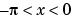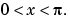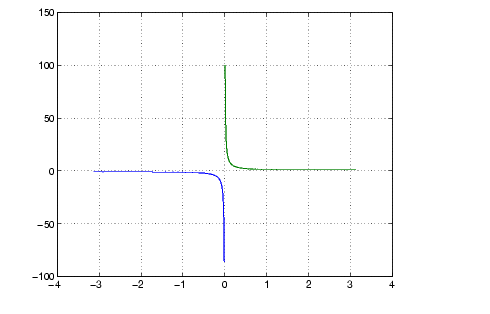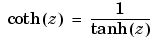MATLAB Function Referencecoth

Hyperbolic cotangent

Syntax

• ```Y = coth(X)
```

Description

The `coth` function operates element-wise on arrays. The function's domains and ranges include complex values. All angles are in radians.

```Y = coth(X) ``` returns the hyperbolic cotangent for each element of `X.`

Examples

Graph the hyperbolic cotangent over the domainsand• ```x1 = -pi+0.01:0.01:-0.01;
x2 = 0.01:0.01:pi-0.01;
plot(x1,coth(x1),x2,coth(x2)), grid on```

Definition

The hyperbolic cotangent can be defined as

•Algorithm

`coth` uses FDLIBM, which was developed at SunSoft, a Sun Microsystems, Inc. business, by Kwok C. Ng, and others. For information about FDLIBM, see http://www.netlib.org.

See Also

`acot`, `acoth`, `cot`

© 1994-2005 The MathWorks, Inc.